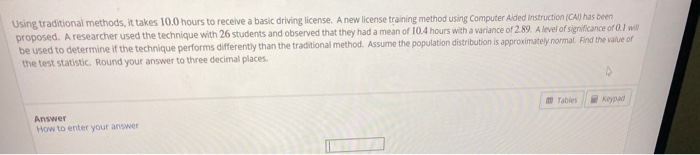Question

Using traditional methods, it takes 96 hours to receive a basic driving license. A new license training method using Computer Aided Instruction (CAI) has been proposed. A researcher used the technique with 290 students and observed that they had a mean of 95 hours. Assume the standard deviation is known to be 8. A level of significance of 0.05 will be used to determine if the technique performs differently than the traditional method. Find the value of the test statistic. Round your answer to 2 decimal places.

Enter the value of the test statistic.

We are given population mean   = 96 hours and population standard deviation = 8 , reseacher studied the sample of 290 students and its mean xbar= 95 .

The sample size is greater than 30 and population standard deviation is known , so we use z test statistic

Z = ( xbar - )/(/√n)

Z = (95-96)/(8/√290)

Z = -2.13

#### Earn Coins

Coins can be redeemed for fabulous gifts.

Similar Homework Help Questions

Using traditional methods, it takes 99 hours to receive a basic driving license. A new license training method using Computer Aided Instruction (CAI) has been proposed. A researcher used the technique with 170 students and observed that they had a mean of 100 hours. Assume the variance is known to be 36. A level of significance of 0.05 will be used to determine if the technique performs differently than the traditional method. Find the value of the test statistic. Round...

Using traditional methods, it takes 96 hours to receive a basic flying license. A new license training method using Computer Aided Instruction (CAI) has been proposed. A researcher used the technique with 150 students and observed that they had a mean of 95 hours. Assume the standard deviation is known to be 88. A level of significance of 0.05 will be used to determine if the technique performs differently than the traditional method. Find the value of the test statistic....

Using traditional methods, it takes 8.5 hours to receive a basic driving license. A new license training method using Computer Aided Instruction (CAI) has been proposed. A researcher used the technique with 16 students and observed that they had a mean of 8.78.7 hours with a variance of 4.00 A level of significance of 0.05 will be used to determine if the technique performs differently than the traditional method. Assume the population distribution is approximately normal. Find the value of...

Using traditional methods, it takes 9898 hours to receive a basic driving license. A new license training method using Computer Aided Instruction (CAI) has been proposed. A researcher used the technique with 7070 students and observed that they had a mean of 9696 hours. Assume the standard deviation is known to be 66. A level of significance of 0.020.02 will be used to determine if the technique performs differently than the traditional method. Find the value of the test statistic....

Using traditional methods, it takes 91 hours to receive a basic driving license. A new license training method using Computer Aided Instruction (CAI) has been proposed. A researcher used the technique with 40 students and observed that they had a mean of 88 88 hours. Assume the standard deviation is known to be 7. A level of significance of 0.1 will be used to determine if the technique performs differently than the traditional method. Find the value of the test...

Using traditional methods, it takes 106 hours to receive a basic driving license. A new license training method using Computer Aided Instruction (CAI) has been proposed. A researcher used the technique with 260 students and observed that they had a mean of 105hours. Assume the variance is known to be 49. A level of significance of 0.02 will be used to determine if the technique performs differently than the traditional method. Find the value of the test statistic. Round your...

Using traditional methods, it takes 8.38.3 hours to receive a basic driving license. A new license training method using Computer Aided Instruction (CAI) has been proposed. A researcher used the technique with 1212 students and observed that they had a mean of 7.97.9 hours with a variance of 3.243.24. A level of significance of 0.10.1 will be used to determine if the technique performs differently than the traditional method. Assume the population distribution is approximately normal. Find the value of...

Using traditional methods it takes 106.0 hours to receive a basic driving license. A new license training method using Computer Aided Instruction (CAI) has been proposed. A researcher used the technique on 60 students and observed that they had a mean of 107.0 hours. Assume the variance is known to be 49.00. Is there evidence at the 0.02 level that the technique performs differently than the traditional method?

Using traditional methods, it takes 9.9 hours to receive a basic driving license. A new license training method using Computer Aided Instruction (CAI) has been proposed. A researcher used the technique with 13 students and observed that they had a mean of 9.8 hours with a standard deviation of 1.5. A level of significance of 0.05 will be used to determine if the technique performs differently than the traditional method. Assume the population distribution is approximately normal. State the null...Using traditional methods, it takes 10.0 hours to receive a basic driving license. A new license training method using Computer Aided instruction (CAI) has been proposed. A researcher used the technique with 26 students and observed that they had a mean of 10.4 hours with a variance of 2.89. A level of significance of Owl be used to determine if the technique performs differently than the traditional method. Assume the population distribution is approximately normal Find the value of the...